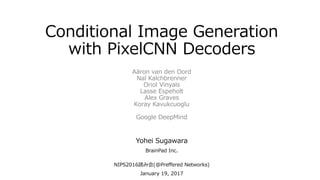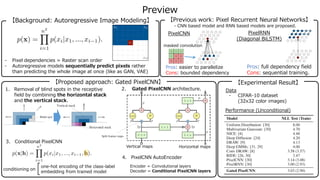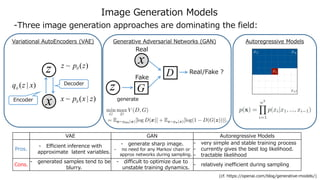Successfully reported this slideshow.

# Conditional Image Generation with PixelCNN Decoders

14

Share×
1 of 21
1 of 21

# Conditional Image Generation with PixelCNN Decoders

14

Share

NIPS2016読み会ポスター発表資料

NIPS2016読み会ポスター発表資料

## More Related Content

### Related Books

Free with a 30 day trial from Scribd

See all

### Related Audiobooks

Free with a 30 day trial from Scribd

See all

### Conditional Image Generation with PixelCNN Decoders

1. 1. Conditional Image Generation with PixelCNN Decoders Yohei Sugawara BrainPad Inc. NIPS2016読み会(@Preferred Networks) January 19, 2017 Aäron van den Oord Nal Kalchbrenner Oriol Vinyals Lasse Espeholt Alex Graves Koray Kavukcuoglu Google DeepMind
2. 2. Preview - Pixel dependencies = Raster scan order - Autoregressive models sequentially predict pixels rather than predicting the whole image at once (like as GAN, VAE) PixelCNN PixelRNN (Diagonal BiLSTM) Pros: easier to parallelize Cons: bounded dependency masked convolution 【Background: Autoregressive Image Modeling】 【Previous work: Pixel Recurrent Neural Networks】 - CNN based model and RNN based models are proposed. Pros: full dependency field Cons: sequential training. 【Proposed approach: Gated PixelCNN】 Vertical maps Horizontal maps one-hot encoding of the class-label embedding from trained model conditioning on 4. PixelCNN AutoEncoder Encoder = Convolutional layers Decoder = Conditional PixelCNN layers 3. Conditional PixelCNN 2. Gated PixelCNN architecture.1. Removal of blind spots in the receptive field by combining the horizontal stack and the vertical stack. 【Experimental Result】 Data - CIFAR-10 dataset (32x32 color images) Performance (Unconditional)
3. 3. Image Generation Models -Three image generation approaches are dominating the field: Variational AutoEncoders (VAE) Generative Adversarial Networks (GAN) z x )(~ zpz θ )|(~ zxpx θ Decoder Encoder )|( xzqφ x z Real D G Fake Real/Fake ? generate Autoregressive Models (cf. https://openai.com/blog/generative-models/) VAE GAN Autoregressive Models Pros. - Efficient inference with approximate latent variables. - generate sharp image. - no need for any Markov chain or approx networks during sampling. - very simple and stable training process - currently gives the best log likelihood. - tractable likelihood Cons. - generated samples tend to be blurry. - difficult to optimize due to unstable training dynamics. - relatively inefficient during sampling
4. 4. Autoregressive Image Modeling - Autoregressive models train a network that models the conditional distribution of every individual pixel given previous pixels (raster scan order dependencies). ⇒ sequentially predict pixels rather than predicting the whole image at once (like as GAN, VAE) - For color image, 3 channels are generated successive conditioning, blue given red and green, green given red, and red given only the pixels above and to the left of all channels. R G B
5. 5. Previous work: Pixel Recurrent Neural Networks.  “Pixel Recurrent Neural Networks” got best paper award at ICML2016.  They proposed two types of models, PixelRNN and PixelCNN (two types of LSTM layers are proposed for PixelRNN.) PixelCNNPixelRNN masked convolution Row LSTM Diagonal BiLSTM PixelRNN PixelCNN Pros. • effectively handles long-range dependencies ⇒ good performance Convolutions are easier to parallelize ⇒ much faster to train Cons. • Each state needs to be computed sequentially. ⇒ computationally expensive Bounded receptive field ⇒ inferior performance Blind spot problem (due to the masked convolution) needs to be eliminated. • LSTM based models are natural choice for dealing with the autoregressive dependencies. • CNN based model uses masked convolution, to ensure the model is causal. 11w 12w 13w 21w 22w 23w 31w 32w 33w 
6. 6. Details of “Masked Convolution” & “Blind Spot”  To generate next pixel, the model can only condition on the previously generated pixels.  Then, to make sure CNN can only use information about pixels above and to the left of current pixel, the filters of the convolution need to be masked. Case 1D  Right figure shows 5x1 convolutional filters after multiplying them by mask.  The filters connecting the input layer to the first hidden layer are in this case multiplied by m=(1,1,0,0,0), to ensure the model is causal. (cf. Generating Interpretable Images with Contollable Structure, S.Reed et.al., 2016) 5x1 filter m=(1,1,0,0,0) Case 2D (ex. text, audio, etc) (ex. image)  In case of 2D, PixelCNNs have a blind spot in the receptive field that cannot be used to make predictions.  Rightmost figure shows the growth of the masked receptive field. (3 layered network with 3x3 conv filters) 5x5 filter 3x3 filter, 3 layered
7. 7. Proposed approach: Gated PixelCNN  In this paper, they proposed the improved version of PixelCNN.  Major improvements are as follows. 1. Removal of blind spots in the receptive field by combining the horizontal stack and the vertical stack. 2. Replacement of the ReLu activations between the masked convolutions in the original PixelCNN with the gated activation unit. 3. Given a latent vector, they modeled the conditional distribution of images, conditional PixelCNN. a. conditioning on class-label b. conditioning on embedding from trained model 4. From a convolutional auto-encoder, they replaced the deconvolutional decoder with conditional PixelCNN, named PixelCNN Auto-Encoders
8. 8. First improvement: horizontal stack and vertical stack  The removal of blind spots in the receptive field are important for PixelCNN’s performance, because the blind spot can cover as much as a quarter of the potential receptive field.  The vertical stack conditions on all rows above the current row.  The horizontal stack conditions on current row. - Details about implementation techniques are described below.
9. 9. Second improvement: Gated activation and architecture  Gated activation unit:  Single layer block of a Gated PixelCNN (σ: sigmoid, k: number of layer, ⦿: element-wise product, *: convolutional operator) - Masked convolutions are shown in green. - Element-wise operations are shown in red. - Convolutions with Wf, Wg are combined into a single operation shown in blue. 𝑣 𝑣′ ℎ ℎ′ 𝑣 = vertical activation maps ℎ = horizontal activation maps inth intv
10. 10. Details of Gated PixelCNN architecture  Break down operations into four steps. ① Calculate vertical feature maps … n×n convolutions are calculated with gated activation. Input: ( = input image if 1st layer) Output: 𝑣 𝑣′ ℎ ℎ′ 𝑣𝑖𝑛𝑡 ℎ𝑖𝑛𝑡 𝑣 𝑣′ Feature map 3x3 masked filters receptive field (1,1,1,1) zero padding receptive field (1,0,1,1) zero padding 2x3 filtersFeature map Two types of equivalent implementation: In this case, (i, j)th pixel depends on (i, j+k)th (future) pixels Next problem: Solution: Shift down vertical feature maps when to feed into horizontal stack. (ex. n=3)
11. 11. 0 0 0 … 0 0 𝑣 𝑣′ ℎ ℎ′ 𝑣𝑖𝑛𝑡 ℎ𝑖𝑛𝑡 Details of Gated PixelCNN architecture ② Feed vertical maps into horizontal stack 1. n x n masked convolution 2. shifting down operation (as below) 3. 1 x 1 convolution Input: ( = input image if 1st layer) Output: 𝑣 𝑣𝑖𝑛𝑡 Shift down vertical feature maps when to feed into horizontal stack. 1. Add zero padding on the top 2. Crop the bottom  Left operations can be interpreted as below. ensure causalityviolate causality Feature map Feature map
12. 12. Details of Gated PixelCNN architecture 𝑣 𝑣′ ℎ ℎ′ 𝑣𝑖𝑛𝑡 ℎ𝑖𝑛𝑡 Feature map 1x3 masked filters receptive field (0,0,1,1) zero padding receptive field (0,0,1,0) zero padding 1x2 filtersFeature map Two types of equivalent implementation: Next problem: ➢ Mask ‘A’ vs Mask ‘B’ ③ Calculate horizontal feature maps … 1×n convolutions are calculated with gated activation. (vertical maps are added before activation.) Input: , (input image if 1st layer) Output: ℎ inth intv - Mask ‘A’ (restrict connection from itself) is applied to only to the first convolution. - Mask ‘B’ (allow connection from itself) is applied to all the subsequent convolution. (ex. n=3)
13. 13. 𝑣 𝑣′ ℎ ℎ′ Details of Gated PixelCNN architecture ④ Calculate residual connection in horizontal stack. … 1×1 convolutions are calculated without gated activation. then, maps are added to horizontal maps (of layer’s input) Input: , (input image if 1st layer) Output: ℎ ℎ′ inth inth intv ③ Calculate horizontal feature maps - Mask ‘A’ can be implemented as below. (ex. n=3) receptive field (0,0,1,0) zero padding 1x1 filters Feature map  [Convolution] [Crop the right]
14. 14. Output layer and whole architecture  Output layer  Using a softmax on discrete pixel values ([0-255] = 256 way) instead of a mixture density approach. (same approach as PixelRNN)  Although without prior information about the meaning or relations of the 256 color categories, the distributions predicted by the model are meaningful.  Whole architecture (width) x (height) x (channels) … … (width) x (height) x p (#feature maps) Gated PixelCNN layer output Input Additional 1x1 conv layers
15. 15. original conditional Model Gated activation unit Third improvements: conditional PixelCNN & PixelCNN AutoEncoder  they modeled the conditional distribution by adding terms that depend on h to the activations before the nolinearities  coniditional PixelCNN  PixelCNN AutoEncoder  From a convolutional auto-encoder, they replaced the deconvolutional decoder with conditional PixelCNN Encoder:Convolution layers Decoder:Deconvolution layers ⇒ conditional PixelCNN layers
16. 16. Experimental Results (Unconditional)  Data: CIFAR-10 dataset  Score: Negative log-likelihood score (bits/dim)  Gated PixelCNN outperforms the PixelCNN by 0.11 bits/dim, which has a very significant effect on the visual quality, and close to the performance of PixelRNN  Data: ImageNet dataset  Gated PixelCNN outperforms PixelRNN.  Achieve similar performance to the PixelRNN in less than half the training time.
17. 17. Experimental Results (Conditional)  Coniditioning on ImageNet classes  Given a one-hot encoding hi, for the i-th class, model )|( ihxp  Coniditioning on Portrait Embeddings (part of results.)  Embeddings are took from top layer of a conv network trained on a large database of portraits from Flickr images.  After the supervised net was trained, {x:image, h:embedding} tuples are taken and trained conditional PixelCNN to model  Given a new image of a person that was not in the training set, they computed h and generate new portraits of same person. )|( hxp  And experimented with reconstructions conditioned on linear interpolations between embeddings of pairs of images.
18. 18. Experimental Results (PixelCNN Auto-Encoder)  Data: 32x32 ImageNet patches (Left to right: original image, reconstruction by auto-encoder, conditional samples from PixelCNN auto-encoder) (m: dimensional bottleneck)
19. 19. Summary & Reference  Improved PixelCNN:  Same performance as PixelRNN, but faster (easier to parallelize)  Fixed “blind spots” problem  Gated activation units  Conditional Generation:  Conditioned on class-label  Conditioned on portrait embedding  PixelCNN AutoEncoders  Summary  References  Aäron van den Oord et al., “Conditional Image Generation with PixelCNN Decoders”, NIPS 2016  Aäron van den Oord et al., “Pixel Recurrent Neural Networks”, ICML 2016 (Best Paper Award)  S. Reed, A. van den Oord et al., “Generating Interpretable Images with Controllable Structure”, Under review as a conference paper at ICLR 2017
20. 20. Appendix – Progress of research related to this paper  Applied to other domains ➢ “WaveNet: A Generative Model for Raw Audio”, A. van den Oord et al. (DeepMind) ➢ “Video Pixel Networks”, Nal Kalchbrenner, A. van den Oord et al. (DeepMind) ➢ “Language Modeling with Gated Convolutional Networks”, Yann N.Dauphin et al. (Facebook AI Research) ➢ “Generating Interpretable Images with Controllable Structure”, S.Reed, A. van den Oord et al. (Google DeepMind), Under review as a conference paper at ICLR 2017  The conditional probability distribution is modelled by a stack of dilated causal convolutional layers with gated activation units.  The architecture of the generative video model consists of two parts: 1. Resolution preserving CNN encoders 2. PixelCNN decoders  Text-to-image synthesis (generating images from captions and other strcuture) using gated conditional PixelCNN model.  A new language model that replace recurrent connections typically used in RNN with gated temporal convolutions.
21. 21. Appendix – Progress of research related to this paper ➢ “PixelCNN++: A PixelCNN Inplementation with Discretized Logistic Mixture Likelihood and Other Modifications”, Tim Salimans, Andrej Karpathy, et al. (OpenAI), Under review as a conference paper at ICLR 2017  Modifications of Gated PixelCNN model ➢ “PixelVAE: A Latent Variable Model for Natural Images”, Ishaan Gulrajani, Kundan Kumar et al., Under review as a conference paper at ICLR 2017  A number of modifications to the original gated PixelCNN model. 1. Use a discretized logistic mixture likelihood, rather than a 256-way sofmax. 2. Condition on whole pixels, rather than R/G/B sub-pixels. 3. Use downsampling. 4. Introduce additional short-cut connections. (like as U-net) 5. Regularize the model using dropout.  A VAE model with an autoregressive decoder based on PixelCNN.